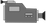Slope

The following videos describe the concept of slope --in context, within an equation, within a data table, and on a graph.

Slope in Context (.mp4)Slope in an Equation (.mp4)Slope in Data Tables (.mp4)Slope_Graph (.mp4)1. Slope in Context2. Slope in an Equation3. Slope on Data Tables4. Slope on a Graph